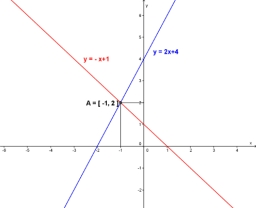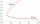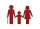# Equations - simple

Solve system of linear equations:

x-2y=6
3x+2y=4

x =  2.5
y =  -1.75

### Step-by-step explanation:

x-2y=6
3x+2y=4

x-2•y=6
3•x+2•y=4

x-2y = 6
3x+2y = 4

x = 5/2 = 2.5
y = -7/4 = -1.75

Our linear equations calculator calculates it.Did you find an error or inaccuracy? Feel free to write us. Thank you!Math student
hi i liked this site it helps me in practicing for my exams and knowledgeMatematik
I understand it is a great site I loved it it help me study it was greatI’d like to say it was great.Tips to related online calculators
Do you have a system of equations and looking for calculator system of linear equations?

## Related math problems and questions:

• EquationsSolve following system of equations: 6(x+7)+4(y-5)=12 2(x+y)-3(-2x+4y)=-44
• Consider 2Consider the following formula: y = 3 ( x + 5 ) ( x - 2 ) Which of the following formulas is equivalent to this one? A. Y=3x2+9x-30 B. Y=x2+3x-10 C. Y=3x2+3x-10 D. Y=3x2+3x-30
• Linear systemSolve this linear system (two linear equations with two unknowns): x+y =36 19x+22y=720
• Two equationsSolve equations (use adding and subtracting of linear equations): -4x+11y=5 6x-11y=-5
• Linear systemSolve a set of two equations of two unknowns: 1.5x+1.2y=0.6 0.8x-0.2y=2
• Non linear eqsSolve the system of non-linear equations: 3x2-3x-y=-2 -6x2-x-y=-7
• Three points 4The line passed through three points - see table: x y -6 4 -4 3 -2 2 Write line equation in y=mx+b form
• Substitutionsolve equations by substitution: x+y= 11 y=5x-25
• Elimination methodSolve system of linear equations by elimination method: 5/2x + 3/5y= 4/15 1/2x + 2/5y= 2/15
• Linsys2Solve two equations with two unknowns: 400x+120y=147.2 350x+200y=144
• Simple equationsSolve system of equations: 5x+3y=5 5x+7y=25
• Intersections 3Find the intersections of the circles x2 + y2 + 6 x - 10 y + 9 = 0 and x2 + y2 + 18 x + 4 y + 21 = 0
• ProveProve that k1 and k2 are the equations of two circles. Find the equation of the line that passes through the centers of these circles. k1: x2+y2+2x+4y+1=0 k2: x2+y2-8x+6y+9=0
• Sphere from tree pointsEquation of sphere with three point (a,0,0), (0, a,0), (0,0, a) and center lies on plane x+y+z=a
• If x=4If x=4 and y=-3, what is the value of the expression x² + 2xy - 4x + 3y + y²?
• Father and sonWhen I was eight years old, my father was 30 years old. Today, my father is three times more than me. How old am I?
• My fatherMy father has a big farm. 6/8 of it were planted with mango trees, 1/2 of the remainder are guava trees and the rest 10 trees are santol trees. What is the number of all trees?